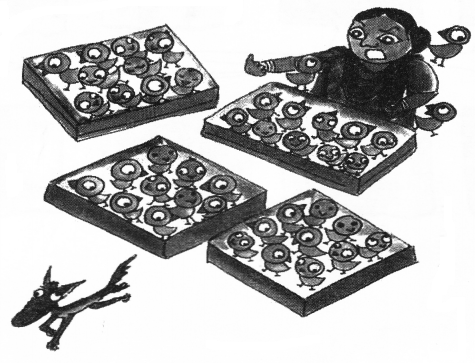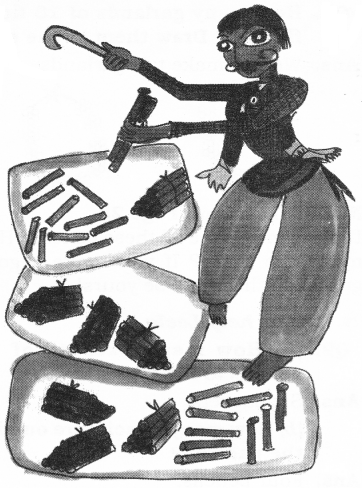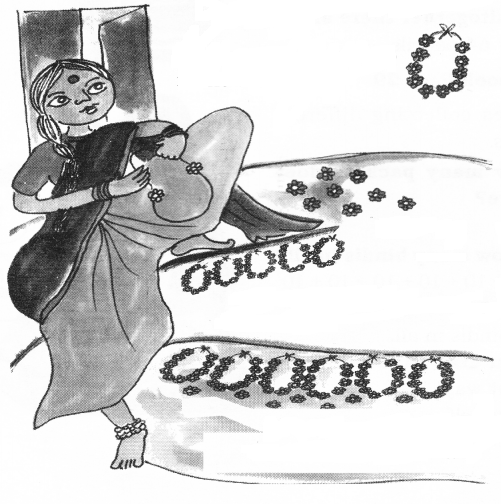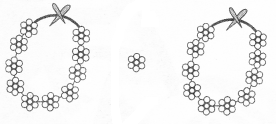NCERT Solutions for Class 2 Maths Chapter 4 Counting in Tens are part of NCERT Solutions for Class 2 Maths. Here we have given NCERT Solutions for Class 2 Maths Chapter 4 Counting in Tens.

 Board CBSE Textbook NCERT Class Class 2 Subject Maths Chapter Chapter 4 Chapter Name Counting in Tens Number of Questions 11 Category NCERT Solutions

## NCERT Solutions for Class 2 Maths Chapter 4 Counting in Tens

NCERT Textbook Page 25
Chickens and the Clever FoxQuestion 1.
In the morning, she counted her chickens.
(a) How many baskets of 10 chickens are there?
(b) How many chickens are there in ail?
(b) 50 + 4 = 54.

Question 2.
In the’evening, she counted the chickens again.(a) There are 4 baskets of 10 chickens.
(b) There are 40 + 3 = 43 chickens in all.
(c) 54 – 43 = 11 chickens have been eaten by the fox.

NCERT Textbook Page 26
How Many are These?
Bhanu collects sticks from the jungle.
He sells them in the market.
He uses 10 sticks to make 1 bundle.Question 3.
3 bundles have ____ sticks.
30.

Question 4.
Now, how many sticks in all are these?
30 + 9 = 39 sticks in all.

Question 5.
4 bundles would have sticks.
40 sticks.

NCERT Textbook Page 27
There is a wedding in Malti’s house.
She is making flower garlands.
She uses 10 flowers to make 1 garland.Question 6.
So there are ______ flowers in all.
58

Question 7.
How many flowers are these?
67 flowers in all.

Question 8.
How many garlands of 10 flowers each can you make using 21 flowers? Draw them in the space below.
We can make two garlands.NCERT Textbook Page 28
Leda, can you see all these pencils? How many are these? If you make a good guess, all these will be yours!
Let us help Leela.Question 9.
(a) How many packets of 10 pencils are there?
(b) How many pencils are outside the packets?
(c) So, altogether there are
(a) Six packets.
(b) Four pencils.
(c) 60 + 4 = 64 pencils.

NCERT Textbook Page 29
Kanak likes collecting different kinds of bindis.Question 10.
(a) How many packets does she have?
(b) So how many bindis in ail?